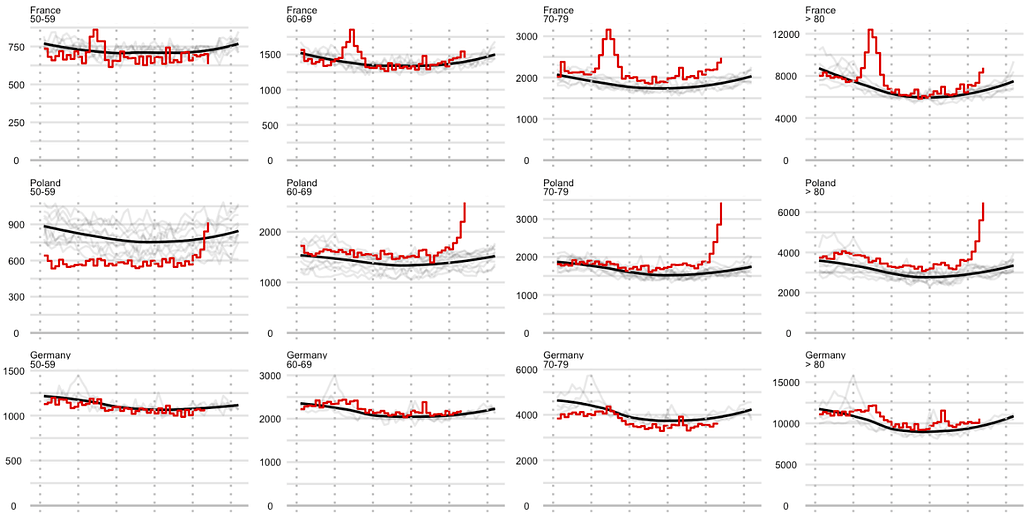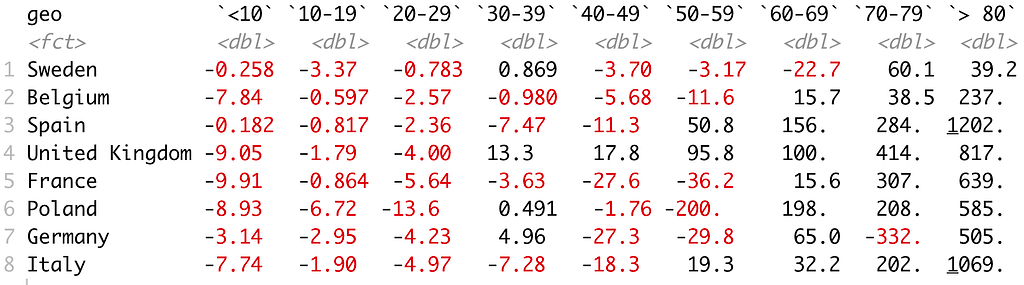[This article was first published on Stories by Przemyslaw Biecek on Medium, and kindly contributed to R-bloggers]. (You can report issue about the content on this page here)
Want to share your content on R-bloggers? click here if you have a blog, or here if you don't.Number of deaths in consecutive weeks. See the second plot for the whole story.

Recently there have been several blog entries showing excessive number of deaths in different countries.
Recently I discovered that in the eurostat database (1) one can find current data on the number of deaths, (2) this number is broken down by age, gender and geographical area, (3) one can use the ‘eurostat’ package to easily read and plot these data.

It turns out that the difference in the number of deaths by age leads to interesting observations.

Read the data from demo_r_mwk_10 table from eurostat.

library(eurostat)
mdata <- get_eurostat("demo_r_mwk_10")
mdata2010 <- mdata[as.character(mdata$time) >= "2010",] Do some cleaning in order to select only interesting age groups, interesting countries and genders (here T stands for Total). age_group <- c("Y_LT10" = "<10", "Y10-19" = "10-19", "Y20-29" = "20-29", "Y30-39" = "30-39", "Y40-49" = "40-49", "Y50-59" = "50-59", "Y60-69" = "60-69", "Y70-79" = "70-79", "Y_GE80" = "> 80") mdata2010 <- mdata2010[mdata2010$age %in% names(age_group), ]
mdata2010$age <- factor(mdata2010$age, levels = names(age_group), labels = age_group)
geo_group <- c("SE" = "Sweden", "BE" = "Belgium", "ES" = "Spain",
"UK" = "United Kingdom", "FR" = "France", "PL" = "Poland",
"DE" = "Germany", "IT" = "Italy")
mdata2010 <- mdata2010[mdata2010$geo %in% names(geo_group), ] mdata2010$geo <- factor(mdata2010$geo, levels = names(geo_group), labels = geo_group) mdata2010 <- mdata2010[mdata2010$sex %in% c("T"), ]
mdata2010$year <- substr(mdata2010$time, 1, 4)
mdata2010$week <- as.numeric(substr(mdata2010$time, 6, 7))

And plot it with ggplot2. Note that we force to have 0 in the plot (geom_hline), there is smoothed average for years 2010–2019 (gem_smooth), this year is presented with a step function because we have weekly aggregates (geom_step) and everything is split into small panels with theme_wrap().

ggplot(mdata2010, aes(week, values, group=paste(sex, year))) +
geom_line(data = mdata2010[mdata2010$year!="2020",], alpha = 0.1) + geom_smooth(data = mdata2010[mdata2010$year!="2020",], se = FALSE, group = 1, color = "black", size=0.6) +
geom_hline(yintercept = 0, color="grey", size=0.5) +
geom_step(data = mdata2010[mdata2010\$year=="2020",], color = "red3") +
facet_wrap(geo~age, scales = "free_y", ncol = 9) + xlim(0,52) + ylab("Number of deaths (eurostat)") +
geom_vline(xintercept = seq(0,50,10), color="grey", lty=3)+
DALEX::theme_ema() + ggtitle("Excessive deaths in 2020 by age\nRed - data for 2020, grey - data for 2010-2019, black - average for 2010-2019")

The results are below. A quick observation: mortality among young people is low and we do not observe excessive deaths below age 50. Note that for most countries we have data till week 44–45.

You can find the code and images presented below on the MOCOS (MOdeling COronavirus Spread) GitHub.The difference between the average number of deaths per week in 2020 and the average number of deaths per week in 2010–2019.

If you are interested in other posts about explainable, fair, and responsible ML, follow #ResponsibleML on Medium.

In order to see more R related content visit https://www.r-bloggers.comPlotting the excessive number of deaths in 2020 by age (with the eurostat package) was originally published in ResponsibleML on Medium, where people are continuing the conversation by highlighting and responding to this story.